# Microwave measurements

## Microwave measurements

A collection of techniques particularly suited for development of devices and monitoring of systems where physical size of components varies from a significant fraction of an electromagnetic wavelength to many wavelengths.

Virtually all microwave devices are coupled together with a transmission line having a uniform cross section. The concept of traveling electromagnetic waves on that transmission line is fundamental to the understanding of microwave measurements.

At any reference plane in a transmission line there are considered to exist two independent traveling electromagnetic waves moving in opposite directions. One is called the forward or incident wave, and the other the reverse or reflected wave. The electromagnetic wave is guided by the transmission line and is composed of electric and magnetic fields with associated electric currents and voltages. Any one of these parameters can be used in considering the traveling waves, but the measurements in the early development of microwave technology made principally on the voltage waves led to the custom of referring only to voltage. One parameter in very common use is the voltage reflection coefficient Γ, which is related to the incident, Vi, and reflected, Vr voltage waves by Eq. (1). (1)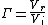#### Impedance

The voltage reflection coefficient Γ is related to the impedance terminating the transmission line and to the impedance of the line itself. If a wave is launched to travel in only one direction on a uniform reflectionless transmission line of infinite length, there will be no reflected wave. The input impedance of this infinitely long transmission line is defined as its characteristic impedance Z0. An arbitrary length of transmission line terminated in an impedance Z0 will also have an input impedance Z0. See Electrical impedance

If the transmission line is terminated in the arbitrary complex impedance load ZL, the complex voltage reflection coefficient ΓL at the termination is given by Eq. (2).

(2)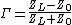Even when there is no unique expression for ZL and Z0 such as in the case of hollow uniconductor waveguides, the voltage reflection coefficient Γ has a value because it is simply a voltage ratio. In general, the measurement of microwave impedance is the measurement of Γ. Both amplitude and phase of Γ can be measured by direct probing of the voltage standing wave set up along a transmission line by the two opposed traveling waves, but this is a slow technique. Directional couplers have been used for many years to perform much faster swept frequency measurement of the magnitude of Γ, and more recently the use of automatic network analyzers under computer control has made possible rapid, accurate measurements of amplitude and phase of Γ over very broad frequency ranges.

#### Power

A required increase in microwave power is expensive whether it be the output from a laboratory signal generator, the power output from a power amplifier on a satellite, or the cooking energy from a microwave oven. To minimize this expense, absolute power must be measured. Most techniques involve conversion of the microwave energy to heat energy which, in turn, causes a temperature rise in a physical body. This temperature rise is measured and is approximately proportional to the power dissipated. The whole device can be calibrated by reference to low-frequency electrical standards and application of appropriate corrections. See Radiometry

The power sensors are simple and can be made to have a very broad frequency response. A power meter can be connected directly to the output of a generator to measure available power PA, or a directional coupler may be used to permit measurement of a small fraction of the power actually delivered to the load.

#### Scattering coefficients

While the measurement of absolute power is important, there are many more occasions which require the measurement of relative power which is equivalent to the magnitude of voltage ratio and is related to attenuation. Also there arises frequently the need to measure the relative phase of two voltages. Measurement systems having this capability are referred to as vector network analyzers, and they are used to measure scattering coefficients of multi-port devices. The concept of scattering coefficients is an extension of the voltage reflection coefficient applied to devices having more than one port. The most simple is a two-port. Its characteristics can be specified completely in terms of a 2 × 2 scattering matrix, the coefficients of which are indicated in the illustration. The incident voltage at the reference plane of each port is defined as a, and the reflected voltage is b. Voltages a and b are related by matrix equation (3), where (Snm) is the scattering matrix of the junction. Writing Eq. (3) out for a two-port device gives Eqs. (4)

(3)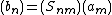(4)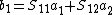(5)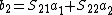and (5). Examination of Eq. (4) shows, for example, that S11 is the voltage reflection coefficient looking into port 1 if port 2 is terminated with a Z0 load (a2 = 0).

#### Heterodyne

The heterodyne principle is used for scalar attenuation measurements because of its large dynamic range and for vector network analysis because of its phase coherence. The microwave signal at frequency fs is mixed with a microwave local oscillator at frequency fLO, in a nonlinear mixer. The mixer output signal at frequency fs - fLO is a faithful amplitude and phase reproduction of the original microwave signal but is at a low, fixed frequency so that it can be measured simply with low-frequency techniques. One disadvantage of the heterodyne technique at the highest microwave frequencies is its cost. Consequently, significant effort has been expended in development of multiport network analyzers which use several simple power detectors and a computer analysis approach which allows measurement of both relative voltage amplitude and phase with reduced hardware cost.

#### Noise

Microwave noise measurement is important for the communications field and radio astronomy. The measurement of thermal noise at microwave frequencies is essentially the same as low-frequency noise measurement, except that there will be impedance mismatch factors which must be carefully evaluated. The availability of broadband semiconductor noise sources having a stable, high, noise power output has greatly reduced the problems of source impedance mismatch because an impedance-matching attenuator can be inserted between the noise source and the amplifier under test.

#### Use of computers

The need to apply calculated corrections to obtain the best accuracy in microwave measurement has stimulated the adoption of computers and computer-controlled instruments. An additional benefit of this development is that measurement techniques that are superior in accuracy but too tedious to perform manually can now be considered.

McGraw-Hill Concise Encyclopedia of Physics. © 2002 by The McGraw-Hill Companies, Inc.

## Microwave measurements

A collection of techniques particularly suited for development of devices and monitoring of systems where physical size of components varies from a significant fraction of an electromagnetic wavelength to many wavelengths.

Virtually all microwave devices are coupled together with a transmission line having a uniform cross section. The concept of traveling electromagnetic waves on that transmission line is fundamental to the understanding of microwave measurements.

At any reference plane in a transmission line there are considered to exist two independent traveling electromagnetic waves moving in opposite directions. One is called the forward or incident wave, and the other the reverse or reflected wave. The electromagnetic wave is guided by the transmission line and is composed of electric and magnetic fields with associated electric currents and voltages. Any one of these parameters can be used in considering the traveling waves, but the measurements in the early development of microwave technology made principally on the voltage waves led to the custom of referring only to voltage. One parameter in very common use is the voltage reflection coefficient Γ, which is related to the incident, Vi, and reflected, Vr voltage waves by Eq. (1). (1)#### Impedance

The voltage reflection coefficient Γ is related to the impedance terminating the transmission line and to the impedance of the line itself. If a wave is launched to travel in only one direction on a uniform reflectionless transmission line of infinite length, there will be no reflected wave. The input impedance of this infinitely long transmission line is defined as its characteristic impedance Z0. An arbitrary length of transmission line terminated in an impedance Z0 will also have an input impedance Z0.

If the transmission line is terminated in the arbitrary complex impedance load ZL, the complex voltage reflection coefficient ΓL at the termination is given by Eq. (2).

(2)Even when there is no unique expression for ZL and Z0 such as in the case of hollow uniconductor waveguides, the voltage reflection coefficient Γ has a value because it is simply a voltage ratio. In general, the measurement of microwave impedance is the measurement of Γ. Both amplitude and phase of Γ can be measured by direct probing of the voltage standing wave set up along a transmission line by the two opposed traveling waves, but this is a slow technique. Directional couplers have been used for many years to perform much faster swept frequency measurement of the magnitude of Γ, and more recently the use of automatic network analyzers under computer control has made possible rapid, accurate measurements of amplitude and phase of Γ over very broad frequency ranges.

#### Power

A required increase in microwave power is expensive whether it be the output from a laboratory signal generator, the power output from a power amplifier on a satellite, or the cooking energy from a microwave oven. To minimize this expense, absolute power must be measured. Most techniques involve conversion of the microwave energy to heat energy which, in turn, causes a temperature rise in a physical body. This temperature rise is measured and is approximately proportional to the power dissipated. The whole device can be calibrated by reference to low-frequency electrical standards and application of appropriate corrections.

The power sensors are simple and can be made to have a very broad frequency response. A power meter can be connected directly to the output of a generator to measure available power PA, or a directional coupler may be used to permit measurement of a small fraction of the power actually delivered to the load.

#### Scattering coefficients

While the measurement of absolute power is important, there are many more occasions which require the measurement of relative power which is equivalent to the magnitude of voltage ratio and is related to attenuation. Also there arises frequently the need to measure the relative phase of two voltages. Measurement systems having this capability are referred to as vector network analyzers, and they are used to measure scattering coefficients of multi-port devices. The concept of scattering coefficients is an extension of the voltage reflection coefficient applied to devices having more than one port. The most simple is a two-port. Its characteristics can be specified completely in terms of a 2 × 2 scattering matrix, the coefficients of which are indicated in the illustration. The incident voltage at the reference plane of each port is defined as a, and the reflected voltage is b. Voltages a and b are related by matrix equation (3), where (Snm) is the scattering matrix of the junction. Writing Eq. (3) out for a two-port device gives Eqs. (4)

(3)(4)(5)and (5). Examination of Eq. (4) shows, for example, that S11 is the voltage reflection coefficient looking into port 1 if port 2 is terminated with a Z0 load (a2 = 0).

#### Heterodyne

The heterodyne principle is used for scalar attenuation measurements because of its large dynamic range and for vector network analysis because of its phase coherence. The microwave signal at frequency fs is mixed with a microwave local oscillator at frequency fLO, in a nonlinear mixer. The mixer output signal at frequency fs - fLO is a faithful amplitude and phase reproduction of the original microwave signal but is at a low, fixed frequency so that it can be measured simply with low-frequency techniques. One disadvantage of the heterodyne technique at the highest microwave frequencies is its cost. Consequently, significant effort has been expended in development of multiport network analyzers which use several simple power detectors and a computer analysis approach which allows measurement of both relative voltage amplitude and phase with reduced hardware cost.

#### Noise

Microwave noise measurement is important for the communications field and radio astronomy. The measurement of thermal noise at microwave frequencies is essentially the same as low-frequency noise measurement, except that there will be impedance mismatch factors which must be carefully evaluated. The availability of broadband semiconductor noise sources having a stable, high, noise power output has greatly reduced the problems of source impedance mismatch because an impedance-matching attenuator can be inserted between the noise source and the amplifier under test.

#### Use of computers

The need to apply calculated corrections to obtain the best accuracy in microwave measurement has stimulated the adoption of computers and computer-controlled instruments. An additional benefit of this development is that measurement techniques that are superior in accuracy but too tedious to perform manually can now be considered.

McGraw-Hill Concise Encyclopedia of Engineering. © 2002 by The McGraw-Hill Companies, Inc.
References in periodicals archive ?
Accurate microwave measurements are becoming increasingly necessary in harsh and hard-to-reach environments spanning a wide range of conditions: day or night, rain or shine, hot or cold; aboard a ship, in an aircraft, or in a vehicle.
The configuration of radiometers was initially chosen to mimic those found aboard, or planned for, major European satellites, such as the Meteorological Operational satellites (MetOp), as well as microwave measurements made by the Special Sensor Microwave Imager (SSM/I), Global Precipitation Measurement (GPM) Microwave Imager (GMI), Advanced Microwave Sounding Unit A and B (AMSU-A,B), and Advanced Microwave Scanning Radiometer (AMSR).
The session "Cellular-Level Microwave Measurements" reviewed advances in cellular characterization via interaction with RF/ microwave fields.
Indirect microwave holographic imaging differs from conventional microwave imaging techniques in that it does not require the use of expensive vector measurement equipment to obtain the complex field scattered from the object under imaging, but mathematically recovers it from low-cost intensity-only scalar microwave measurements. This simplifies the hardware implementation and significantly reduces the cost of the imaging system.
Very precise microwave measurements between two spacecraft, named Ebb and Flow, were used to map gravity with high precision and high spatial resolution.
Better yet, FieldFox delivers Agilent-quality microwave measurements - wherever our customers need to go."
Immediately after making the microwave measurements at the marked locations the board samples were cut up at the marked measurement locations and immediately weighed in order to allow determination of the MC at each measurement location.
Workshops will be held Sunday, Monday and Friday, and the ARFTG Microwave Measurements Conference will be held on Friday.
NIST is creating a "High-Speed Measurement Laboratory." This laboratory will develop calibratable highspeed electrical and optoelectronic measurements for next-generation optical telecommunications, electrical phase standards for nonlinear and other microwave measurements, and high-speed digital integrated circuits.
Microwave measurements can provide a moisture measurement independent of a product's bulk density.
Preliminary results suggest low-level microwave measurements may lead to development of instruments that growers could use to pinpoint ideal harvesting times.

Site: Follow: Share:
Open / Close